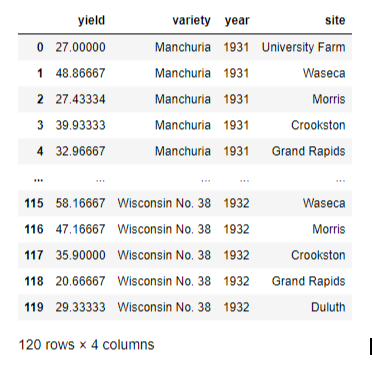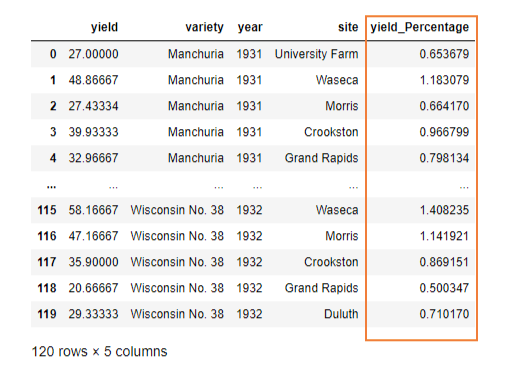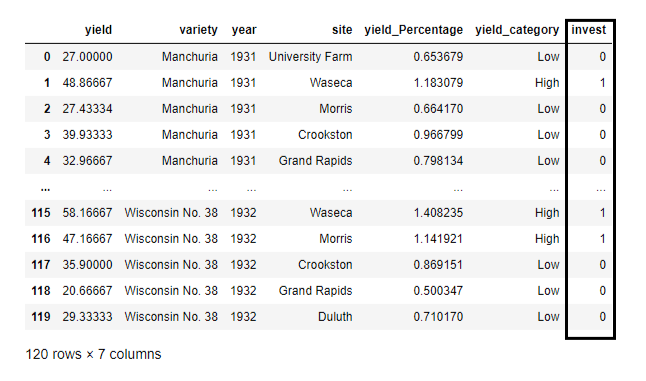top of page

# Lambda function reduces python scripting lines by 80%

In Python, traditionally the functions are declared with the def keyword, while anonymous functions are defined without a name using the lambda keyword.

The syntax of a Lambda function is - lambda arguments: expression

Lambda functions can take any number of parameters but can only execute one expression. We use lambda functions when we require a nameless function.

At first, Lambda functions seem difficult to grasp. They are brief in length yet can be a challenge for a beginner. So, in this blog, you'll discover the potential of lambda functions in Python and how to apply them to fundamental list and data frame operations.

Let us first load the pandas library and a sample dataset to work on:

>>> import pandas as pd

>>> from vega_datasets import data

>>> df = data.barley()

>>> df

Output:## List Operations

>>> site_names = df['site'].unique().tolist()

Traditionally, we use for loops to iterate through a list of elements and apply simple functions. But these for loops can be inconvenient, making the Python code big and untidy.

Let us see an example of a for loop and how we can efficiently obtain similar results through Lambda.

>>> for i in site_names:

>>> i = ''.join(i.split())

>>> i = i.lower()

>>> print(i)

Output:1. Example using Map()

The map() method uses a lambda function and a List and performs the lambda function to all the elements and returns a new List.

>>> a = site_names

>>> b = list(map(lambda x: ''.join(x.split()).lower(), a))

>>> print(b)

Output:2. Example using Filter()

The filter() method uses a lambda function and a List and performs the lambda function to all the elements while filtering the data.

>>> yield_list = df['yield'].tolist()

>>> sub_list = list(filter(lambda x: x > 50, yield_list))

>>> sub_list

Output:3. Example using Reduce()

Using the Reduce() function, the function described by lambda is applied to the first two elements and the result is stored. Thereafter, the function is next applied to the result and third element, and so on. Finally, the list is reduced to a single value at the end.

>>> from functools import reduce

>>> reduce(lambda a,b: a if (a > b) else b, sub_list)

Output:## Dataframe Operations

1. Add a new column by applying function on an existing column using Dataframe.assign()

>>> df = df.assign(yield_Percentage = lambda x: (x['yield']/df['yield'].sum()) * 100)

>>> df

Output:Here, we created a new column ‘yield_Percentage’, and populated it by converting the yield values to percentages.

2. Add a new column using if-else on an existing column using Dataframe.apply()

>>> df['yield_category'] = df['yield'].apply(lambda x: 'Low' if x < 40 else 'High')

>>> df

Output:Here, we created a new column ‘yield_category’ and using an if-else condition on the column ‘yield, assigned ‘Low’ if the yield is less than 40 units or else ‘High’.

3. Iterating over dataframe using Dataframe.apply()

Similar to the Map() function, the Apply() method takes a function as input and applies it to the entire dataframe.

First, we define a function:

>>> def filtering(site, yield_Percentage):

>>> if(site in ['University Farm', 'Waseca', 'Morris']) and yield_Percentage > 1:

>>> return 1

>>> else:

>>> return 0

Secondly, the lambda function is used to iterate across the rows of the dataframe. For every row, we feed the ‘year’, ‘site’, and the ‘yield_Percentage’ column to the filtering function. Finally, axis=0 or axis=1 is mentioned to specify whether the operation is to be applied to the columns or rows, respectively.

>>> df["invest"] = df.apply(lambda row: filtering(row["site"], row["yield_Percentage"]), axis=1)

>>> df

Output:Here, we created a new column ‘invest’ based on the function ‘filtering’ where value 1 is assigned to the rows where yield percentage in the sites 'University Farm', 'Waseca', 'Morris' is more than 1, and otherwise 0.

69 views

Get Started with Listing of your Bug Bounty Program

bottom of page